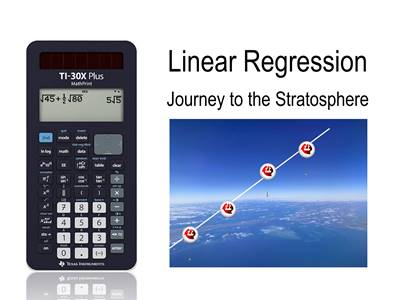•# NSW: Linear Regression Journey

by Texas Instruments#### Objectives

• Fit a Least Squares Regression line
• Interpret the intercept and gradient
• Extrapolate and Interpolate
• Understand Least Squares method

#### Vocabulary

• Least Squares Regression
• Independent variable
• Dependent variable
• Mean (average)
• Extrapolate
• Interpolate